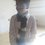I came across this question in a book.

Let $$x$$ and $$a$$ stand for distance. Is $\int\frac{dx}{\sqrt{a^{2}-x^{2}}}=\frac{1}{a}\sin^{-1}\frac{a}{x}$ dimensionally correct?

And this is a solution I found.

Dimension of the left side $=\int\frac{dx}{\sqrt{a^{2}-x^{2}}}=\int\frac{L}{\sqrt{L^{2}-L^{2}}}=[L^{0}]$

Dimension of the right side $=\frac{1}{a}\sin^{-1}\frac{a}{x}=[L^{-1}]$

So, the dimension of $\int\frac{dx}{\sqrt{a^{2}-x^{2}}}\neq\frac{1}{a}\sin^{-1}\frac{a}{x}$

So, the equation is dimensionally incorrect.

Could someone explain me how $\int\frac{L}{\sqrt{L^{2}-L^{2}}}=[L^{0}]$? Shouldn't the denominator be zero or something?Note by Omkar Kulkarni
5 years, 2 months ago

This discussion board is a place to discuss our Daily Challenges and the math and science related to those challenges. Explanations are more than just a solution — they should explain the steps and thinking strategies that you used to obtain the solution. Comments should further the discussion of math and science.

When posting on Brilliant:

• Use the emojis to react to an explanation, whether you're congratulating a job well done , or just really confused .
• Ask specific questions about the challenge or the steps in somebody's explanation. Well-posed questions can add a lot to the discussion, but posting "I don't understand!" doesn't help anyone.
• Try to contribute something new to the discussion, whether it is an extension, generalization or other idea related to the challenge.

MarkdownAppears as
*italics* or _italics_ italics
**bold** or __bold__ bold
- bulleted- list
• bulleted
• list
1. numbered2. list
1. numbered
2. list
Note: you must add a full line of space before and after lists for them to show up correctly
paragraph 1paragraph 2

paragraph 1

paragraph 2

[example link](https://brilliant.org)example link
> This is a quote
This is a quote
    # I indented these lines
# 4 spaces, and now they show
# up as a code block.

print "hello world"
# I indented these lines
# 4 spaces, and now they show
# up as a code block.

print "hello world"
MathAppears as
Remember to wrap math in $$ ... $$ or $ ... $ to ensure proper formatting.
2 \times 3 $2 \times 3$
2^{34} $2^{34}$
a_{i-1} $a_{i-1}$
\frac{2}{3} $\frac{2}{3}$
\sqrt{2} $\sqrt{2}$
\sum_{i=1}^3 $\sum_{i=1}^3$
\sin \theta $\sin \theta$
\boxed{123} $\boxed{123}$

Sort by:

Remember we're not talking about numbers anymore. We're talking about dimensions. Rules of algebra do not nessecarily apply here. So $[L^2] - [L^2]$ is not nessecarily $0$. Both $x^2$ and $a^2$ have a dimesnion of area. So when we add them or subtact them, the resulting quantity will also have the same dimension of area. (as long as the sum/differnce is not 0 ).

Look at it this way. If two sqaures have an area of $9 m^2$ and $4 m^2$, what is the difference of the areas? $5m^2$. The equation here is $9m^2 - 4m^2 = 5m^2$. Or, if you look at this dimensionally, $[L^2] - [L^2] = [L^2]$

- 5 years, 2 months ago

Oh okay! I overlooked that. Thanks! :D

- 5 years, 2 months ago

i think the answer to your integral is wrong ,the given integral is :-

$\int { \frac { dx }{ \sqrt { { a }^{ 2 }-{ x }^{ 2 } } } } =\arcsin { \frac { x }{ a } } +k$

now recheck the dimensions.

u can recheck for yourself ,just substitute $x=$$a\sin { \theta }$

- 5 years, 2 months ago

Yeah, there's a similar question which has $\sin^{-1}\left[\frac{x}{a}+1\right]$. But this integral is meant to be wrong. Thanks though! But why can you substitute $x=a\sin\theta$?

- 5 years, 2 months ago